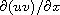Electron. J. Diff. Equ., Vol. 2015 (2015), No. 251, pp. 1-12.

### Lattice Boltzmann method for coupled Burgers equations Yali Duan, Linghua Kong, Xianjin Chen

Abstract:
In this paper, we propose a lattice Boltzmann model for coupled Burgers equations (CBEs). With a proper time-space scale and the Chapman-Enskog expansion, the governing equations are recovered successfully from the lattice Boltzmann equations, and the resulting local equilibrium distribution functions are also obtained. The partial derivativein the model is treated as a source term and discretized with a 2nd-order central difference scheme. Numerical experiments show that the numerical results by the Lattice Boltzmann Method (LBM) either agree well with the corresponding exact solutions or are quite comparable with those available numerical results in the literature.

Submitted May 1, 2015. Published September 25, 2015.
Math Subject Classifications: 65M12, 65M99.
Key Words: Lattice Boltzmann model; equilibrium distribution; coupled Burgers equations; Chapman-Enskog expansion.

Show me the PDF file (466 KB), TEX file, and other files for this article.Yali Duan School of Mathematical Sciences University of Science and Technology of China Hefei, Anhui 230026, China email: ylduan01@ustc.edu.cn Linghua Kong School of Mathematics and Information Science Jiangxi Normal University Nanchang, Jiangxi, 330022, China email: konglh@mail.ustc.edu.cn Xianjin Chen School of Mathematical Sciences University of Science and Technology of China Hefei, Anhui 230026, China email: chenxjin@ustc.edu.cn